# Leray formula

Cauchy–Fantappié formula

A formula for the integral representation of holomorphic functionsof several complex variables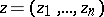,, which generalizes the Cauchy integral formula (see Cauchy integral).

Letbe a finite domain in the complex spacewith piecewise-smooth boundary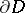and let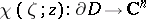be a smooth vector-valued function of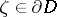with values insuch that the scalar product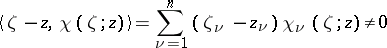everywhere onfor all. Then any functionholomorphic inand continuous in the closed domaincan be represented in the form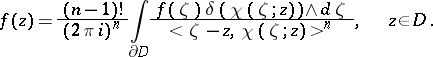(*)

Formula (*) generalizes Cauchy's classical integral formula for analytic functions of one complex variable and is called the Leray formula. J. Leray, who obtained this formula (see ), called it the Cauchy–Fantappié formula. In this formula the differential formsand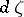are constituted according to the laws: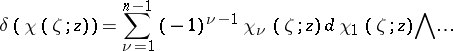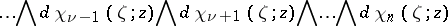and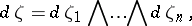whereis the sign of exterior multiplication (see Exterior product). By varying the form of the functionit is possible to obtain various integral representations from formula (*). One should bear in mind that, generally speaking, the Leray integral in (*) is not identically zero whenis outside.